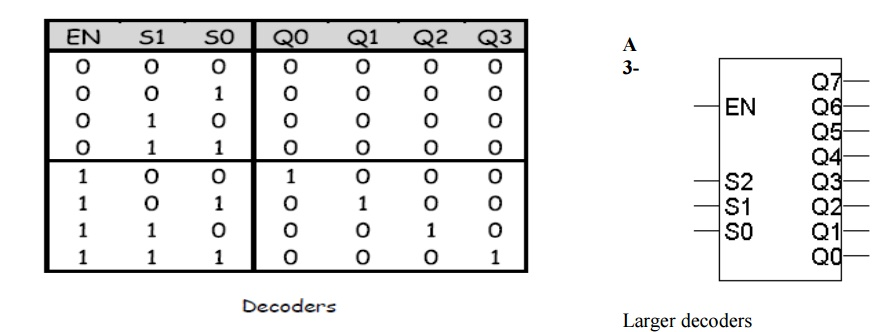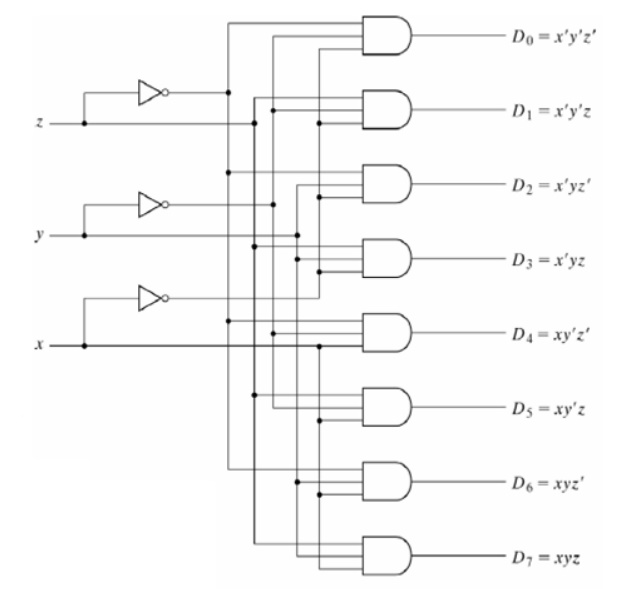Home | | Digital Electronics | Decoders

# Decoders

A combinational circuit that converts binary information from n input lines to a maximum of 2n unique output lines n-to-m-line decoders: generate m (=2n or fewer) minterms of n input variables A n-to-2n decoder takes an n-bit input and produces 2n outputs.

DECODERS:

A combinational circuit that converts binary information from n input lines to a maximum of 2n unique output lines n-to-m-line decoders: generate m (=2n or fewer) minterms of n input variables A n-to-2n decoder takes an n-bit input and produces 2n outputs. The n inputs represent a binary number that determines which of the 2n outputs is uniquely true.

A 2-to-4 decoder operates according to the following truth table. – The 2-bit input is called S1S0, and the four outputs are Q0-Q3. – If the input is the binary number i, then output Qi is uniquely true.

For instance, if the input S1 S0 = 10 (decimal 2), then output Q2 is true, and Q0, Q1, Q3 are all false.

• This circuit “decodes” a binary number into a “one-of-four” code.

Follow the design procedures from last time! We have a truth table, so we can write equations for each of the four outputs (Q0-Q3), based on the two inputs (S0-S1).

• In this case there’s not much to be simplified. Here are the equations:

Q0 = S1’ S0’

Q1 = S1’ S0

Q2 = S1 S0’

Q3 = S1 S0## 2-to-4 decoder

Many devices have an additional enable input, which is used to “activate” or “deactivate” the device. • For a decoder,

– EN=1 activates the decoder, so it behaves as specified earlier. Exactly one of the outputs will be 1.

– EN=0 “deactivates” the decoder. By convention, that means all of the decoder’s outputs are 0.

We can include this additional input in the decoder’s truth table:are similar. Here is a 3-to-8 decoder.

– The block symbol is on the right.

– A truth table (without EN) is below.

– Output equations are at the bottom right.

• Again, only one output is true for any input combinationStudy Material, Lecturing Notes, Assignment, Reference, Wiki description explanation, brief detail
Digital Electronics : Combinational Circuits : Decoders |